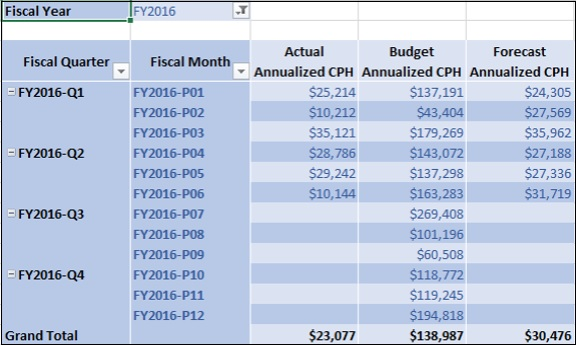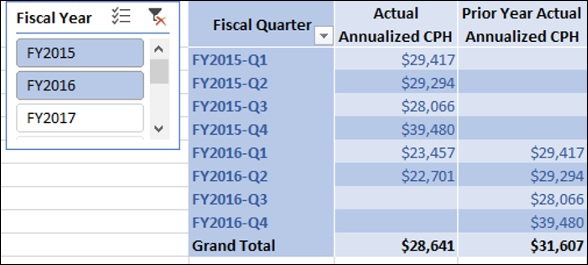# Cost Per Headcount Measures and Analysis

You have learnt about the two major categories of Measures −

• Finance Measures.

The third major category of measures that you will learn is People Cost Measures. Any organization will be interested to know the annualized cost per head. Annualized cost per head represents the cost to the company of having one employee on a full year basis.

To create Cost Per Head measures, you need to first create certain preliminary People Cost Measures. In the Accounts table, you have a column – Sub Class that contains People as one of the values. Hence, you can apply a filter on the Accounts table on the Sub Class column to obtain the filter context onto the Finance Data table to obtain People Cost.

You can use thus obtain People Cost measures and Count of Months measures to create Annualized People Cost measures. You can finally create Annualized Cost Per Head measures from Annualized People Cost measures and Average Head Count measures.

### Creating Actual People Cost Measure

You can create Actual People Cost measure as follows −

Actual People Cost:=CALCULATE('Finance Data'[Actual Sum], FILTER('Finance Data', RELATED(Accounts[Sub Class])="People"))

### Creating Budget People Cost Measure

You can create Budget People Cost measure as follows −

Budget People Cost:=CALCULATE('Finance Data'[Budget Sum], FILTER('Finance Data', RELATED(Accounts[Sub Class])="People"))

### Creating Forecast People Cost Measure

You can create Forecast People Cost measure as follows −

Forecast People Cost:=CALCULATE('Finance Data'[Forecast Sum], FILTER('Finance Data', RELATED(Accounts[Sub Class])="People"))

### Creating Annualized Actual People Cost Measure

You can create Annualized Actual People Cost measure as follows −

Annualized Actual People Cost:=IF([CountOfActualMonths],[Actual People Cost]*12/[CountOfActualMonths],BLANK())

### Creating Annualized Budget People Cost Measure

You can create Annualized Budget People Cost measure as follows −

Annualized Budget People Cost:=IF([CountOfBudgetMonths], [Budget People Cost]*12/[CountOfBudgetMonths],BLANK())

### Creating Annualized Forecast People Cost Measure

You can create Annualized Forecast People Cost measure as follows −

Annualized Forecast People Cost:=IF([CountOfForecastMonths],[Forecast People Cost]*12/[CountOfForecastMonths],BLANK())

### Creating Actual Annualized Cost Per Head Measure

You can create Actual Annualized Cost Per Head (CPH) measure as follows −

Actual Annualized CPH:=IF([Actual Average Headcount], [Annualized Actual People Cost]/[Actual Average Headcount],BLANK() )

### Creating Budget Annualized Cost Per Head Measure

You can create Budget Annualized Cost Per Head (CPH) measure as follows −

### Creating Forecast Annualized Cost Per Head Measure

You can create Forecast Annualized Cost Per Head (CPH) measure as follows −

### Creating Prior Year Actual Annualized Cost Per Head Measure

You can create Prior Year Actual Annualized Cost Per Head (CPH) measure as follows −

Prior Year Actual Annualized CPH:=CALCULATE([Actual Annualized CPH], DATEADD('Date'[Date],-1,YEAR) )

## Analyzing Data with Cost Per Head Measures

Create a Power PivotTable as follows −

• Add the fields Fiscal Quarter and Fiscal Month from Date table to Rows.

• Add the measures Actual Annualized CPH, Budget Annualized CPH, and Forecast Annualized CPH to Columns.

• Add the field Fiscal Year from Date table to Filters.

• Select FY2016 in the Filter.Create another Power PivotTable as follows −

• Add the field Fiscal Quarter from Date table to Rows.

• Add the measures Actual Annualized CPH, and Prior Year Actual Annualized CPH to Columns.

• Insert a Slicer on the field Fiscal Year from Date table.

• Select FY2015 and FY2016 on the Slicer.